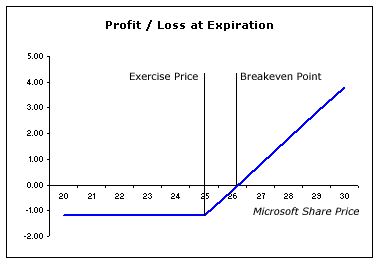TagsToday let’s have a random walk on Wall street to find out how to price an option. An option is a derivative financial contract which derives its value from the performance of another entity such as an asset, index, or interest rate, called the underlying. An option gives the buyer (the owner) the right, but not the obligation, to buy or sell an underlying asset or instrument at a specified strike price on or before a specified date. The buyer pays a premium to the seller for this right.

An option which conveys to the owner the right to buy something at a specific price (called strike) in a future date (called expiry date) is referred to as a call. In contrast, a put conveys the right of the owner to sell something at a specific price. The final payoff depends on the strike, the underlying price at expiry date, and the premium. The following diagram shows the possible payoff of a call option at expiration.A reasonable way to determine the present value of an option (i.e. the premium) is discounting its expected value at the risk-free rate. For a call, we have$C(K,T)=e^{-r(T-t)} \int_0^\infty{\max(S-K,0) p_T(S)dS}$
where$K$ is the strike,$T$ is the expiry date,$t$ is as of date,$r$ is the risk-free interest rate, and$p_T(S)$ is risk-neutral density function of the spot at$T$.

But what does risk-neutral mean? We know that today’s price of a claim on a risky amount realized tomorrow will generally differ from its expected value. Most commonly, investors are risk-averse and today’s price is below the expectation, remunerating those who bear the risk. The calculated expected values need to be adjusted for an investor’s risk preferences.
Unfortunately, the discounted rates would vary between investors and an individual’s risk preference is difficult to quantify. But in a complete market with no arbitrage opportunities, one can adjust, once and for all, the probabilities of future outcomes such that they incorporate all investors’ risk premia, and then take the expectation under this new probability distribution, the risk-neutral measure. Under the risk-neutral probabilities all assets have the same expected rate of return, the risk-free rate.

The condition of no-arbitrage is equivalent to the existence of a risk-neutral measure (the first fundamental theorem of asset pricing). An arbitrage opportunity is a way of making money with no initial investment without any possibility of loss. Arbitrage opportunities do exist briefly in real life. But any sensible market model must avoid this type of profit.

We also mentioned “complete market” above. A complete market is one in which the complete set of possible gambles on future states-of-the-world can be constructed with existing assets without friction. The second fundamental theorem of asset pricing states that there exists a unique risk-neutral measure in a complete market. That is, market efficiency implies that there is only one price. On the other hand, in an incomplete market there are a multitude of possible prices for an asset corresponding to different risk-neutral measures. The correct risk-neutral measure should be selected using economic, rather than purely mathematical, arguments.

A common approach to derive the option price is the famous Black–Scholes model. The key idea behind the model is to hedge the option by buying and selling the underlying asset in just the right way to eliminate risk, known as delta hedging. But we will take a different approach today. Back to our price formula$C(K,T)=e^{-r(T-t)} \int_0^\infty{\max(S-K,0) p_T(S)dS}=e^{-r(T-t)} \int_K^\infty{(S-K) p_T(S)dS}$
It is easy to get the price once we know$p_T(S)$. Before figuring it out, we should notice that$C(K,T)=e^{-r(T-t)}(E(S|S>K)-K(1-CDF_{p_T(S)}(K)))$
which gives a nice financial interpretation of call price. That is, the price is an asset-or-nothing call minus a cash-or-nothing call (long an asset-or-nothing call, short a cash-or-nothing call).

To get$p_T(S)$, we start with stock price$S_0$ at today through a stochastic process. In particular, we assume$S_t$ follow a geometric Brownian motion:$dS_t = \mu S_tdt + \sigma S_t dW_t$
where$W_t$ is a Wiener process or Brownian motion,$\mu$ (the percentage drift) and$\sigma$ (the percentage volatility) are constants.

With Itō’s lemma, it is easy to show that the logarithm of$S_t$ follows a Brownian motion with drift$d \ln S_t =(\frac{1}{S_t} \mu S_t-\frac{1}{2} \frac{1}{S_t^2} \sigma ^2 S_t^2)dt+\frac{1}{S_t} \sigma S_t dW_t=(\mu-\frac{1}{2} \sigma^2 )dt+\sigma dW_t$
Integrating it out, we have$d \ln S_t =(\mu - \frac{1}{2}\sigma^2)\int_0^t{dt}+\sigma\int_0^t{dW_t}=\ln S_0+ (\mu-\frac{1}{2} \sigma^2)t+\sigma W_t$
and$S_t=S_0 e^{(\mu-\frac{1}{2} \sigma^2)t+\sigma W_t}$
So$S_t$ follows a log normal distribution$S_t\sim\ln N(\ln S_0+(\mu-\frac{1}{2}\sigma^2)t,t\sigma^2)$.
However we cannot plugin this density into the integral because this is real-world distribution, not risk-neutral distribution.

The trick is to define$\tilde{W}_t=W_t+\frac{\mu-r}{\sigma}t$, where$\frac{\mu-r}{\sigma}t$ is known as the market price of risk. By Girsanov’s theorem, there exists a measure$Q$ under which$\tilde{W}_t$ is a Brownian motion. Easily, we have$dW_t=d\tilde{W}_t-\frac{\mu-r}{\sigma}dt$. Put it back in the original equation$dS_t = r S_tdt + \sigma S_t d\tilde{W}_t$
With it, we can get the risk-neutral density (simply replace$\mu$ with$r$). This can also be used in the Monte Carlo, which samples with the risk-neutral probability.

Finally we can get the price (the Black-Scholes formula) by integration$C(S,T)=S_0\Phi(d_1) - K e^{-r(T-t)}\Phi(d_2)$
where$d_1=\frac{1}{\sigma\sqrt{T-t}}(\ln\frac{S}{K}+(r+\frac{1}{2}\sigma^2)(T-t))$$d_2=d_1-\sigma\sqrt{T-t}$